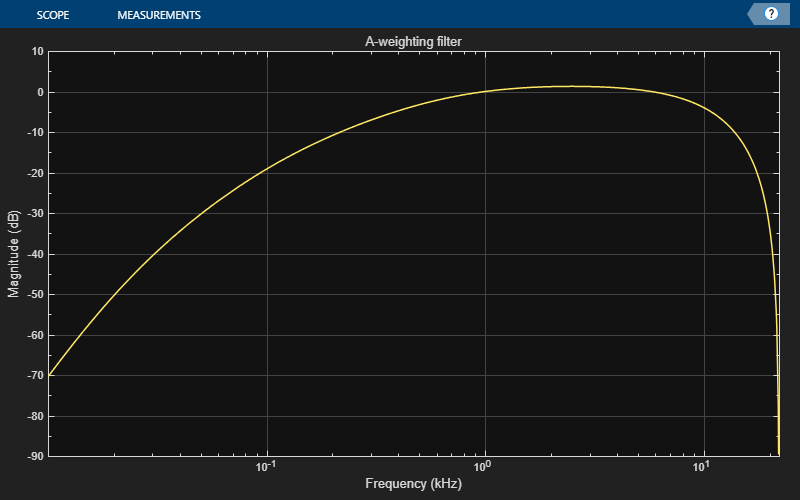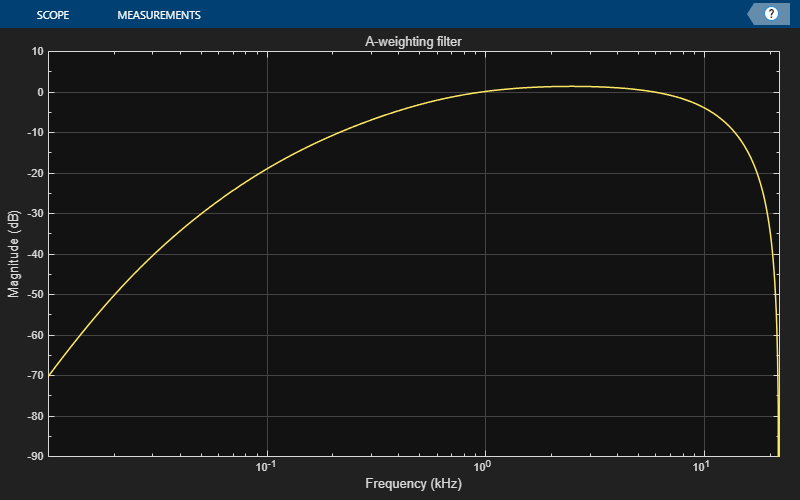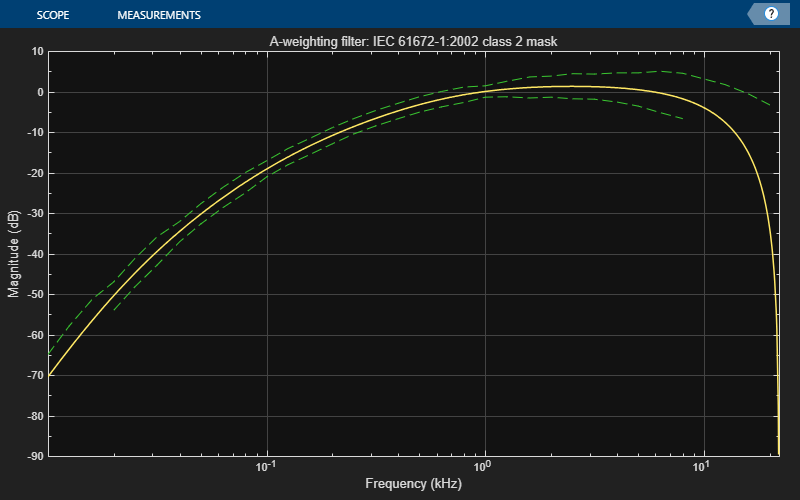Main Content

# visualize

Visualize and validate filter response

## Syntax

``visualize(weightFilt)``
``visualize(weightFilt,N)``
``visualize(___,mType)``

## Description

example

````visualize(weightFilt)` plots the magnitude response of the frequency-weighted filter, `weightFilt`. The plot is updated automatically when properties of the object change.```

example

````visualize(weightFilt,N)` uses an `N`-point FFT to calculate the magnitude response.```

example

````visualize(___,mType)` creates a mask based on the class of filter specified by `mType`, using either of the previous syntaxes.```

## Examples

collapse all

Create an object of the `weightingFilter` System object™ and then plot the magnitude response of the filter.

```weightFilt = weightingFilter; visualize(weightFilt)```Create an object of the `weightingFilter` System object™. Plot a 1024-point frequency representation.

```weightFilt = weightingFilter; visualize(weightFilt,1024)```Create an object of the `weightingFilter` System object™. Visualize the class 2 compliance of the filter design.

```weightFilt = weightingFilter; visualize(weightFilt,'class 2')```## Input Arguments

collapse all

Object of the `weightingFilter` System object™.

Number of DFT bins in frequency-domain representation, specified as a positive scalar. The default is `2048`.

Data Types: `single` | `double`

Type of mask, specified as `'class 1'` or ```'class 2'```.

The mask attenuation limits are defined in the IEC 61672-1:2002 standard. The mask is defined for A-weighting and C-weighting filters only.

• If the mask is green, the design is compliant with the IEC 61672-1:2002 standard.

• If the mask is red, the design breaks compliance.

Note

The pole-zero values defined in the ANSI S1.42-2001 standard are used for designing the A-weighted and C-weighted filters. The pole-zero values are based on analog filters, so the design can break compliance for lower sample rates.

Data Types: `char`

## See Also

### Topics

Introduced in R2016b

## SupportGet trial now